# HBSE 8th Class Maths Solutions Chapter 1 Rational Numbers Ex 1.1

Haryana State Board HBSE 8th Class Maths Solutions Chapter 1 Rational Numbers Ex 1.1 Textbook Exercise Questions and Answers.

## Haryana Board 8th Class Maths Solutions Chapter 1 Rational Numbers Exercise 1.1

Question 1.
Using appropriate properties find:
(i) $$\frac{2}{3} \times \frac{3}{5}+\frac{5}{2}-\frac{3}{5} \times \frac{1}{6}$$
(ii) $$\frac{2}{5} \times\left(\frac{-3}{7}\right)-\frac{1}{6} \times \frac{3}{2}+\frac{1}{14} \times \frac{2}{5}$$
Solution:
(i) $$\frac{2}{3} \times \frac{3}{5}+\frac{5}{2}-\frac{3}{5} \times \frac{1}{6}$$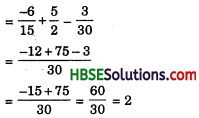2nd Method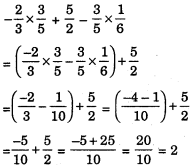3rd Method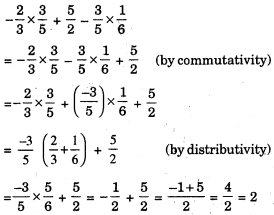(ii) 1st Method
$$\frac{2}{5} \times\left(\frac{-3}{7}\right)-\frac{1}{6} \times \frac{3}{2}+\frac{1}{14} \times \frac{2}{5}$$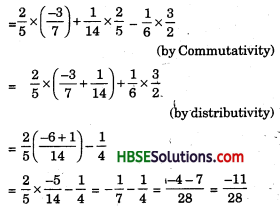2nd Method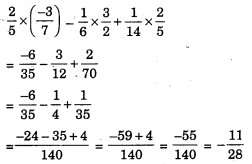Question 2.
Write the additive inverse of each of the following:
(i) $$\frac{2}{8}$$
(ii) $$\frac{-5}{9}$$
(iii) $$\frac{-6}{5}$$
(iv) $$\frac{2}{-9}$$
(v) $$\frac{19}{-6}$$
Solution:
$$\frac{-2}{8}$$ is the additive inverse of $$\frac{2}{8}$$ because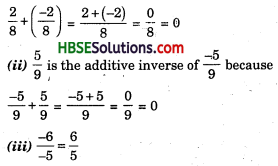$$\frac{-6}{5}$$ is the additive inverse of $$\frac{6}{5}$$ because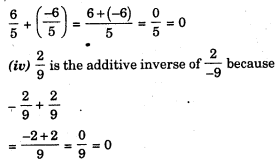Question 3.
Verify that – (-x) = x for
(i) x = $$\frac{11}{15}$$
(ii) x = $$-\frac{13}{17}$$
Solution:
(i) We have, x = $$\frac{11}{15}$$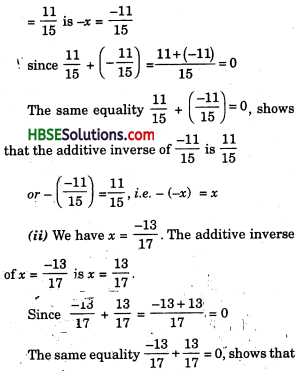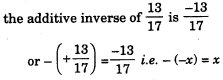Question 4.
Find the multiplicative inverse of the following :
(i) -13
(ii) $$\frac{-13}{19}$$
(iii) $$\frac{1}{5}$$
(iv) $$\frac{-5}{8} \times \frac{-3}{7}$$
(v) -1 × $$\frac{-2}{5}$$
(vi) -1
Solution:
(i) Let the multiplicative inverse of -13 be x.
∴ -13 × x = 1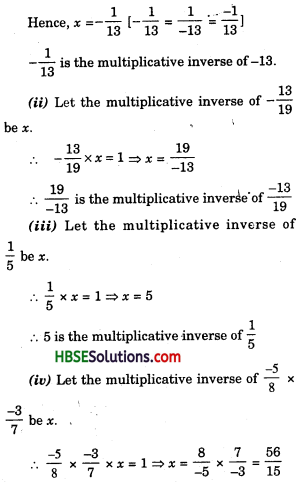(v) Let the multiplicative inverse of -1 × $$\frac{-2}{5}$$ be x.
∴ -1 × $$\frac{-2}{5}$$ × x = 1
⇒ x = $$\frac{1 \times 5}{-1 \times(-2)}$$ = $$\frac{5}{2}$$ or $$\frac{-5}{-2}$$
(vi) The multiplicative inverse of -1 is -1 because -1 × (-1) = 1Question 5.
Name the property under multiplication used in each of the following:
(i) $$\frac{-4}{5} \times 1=1 \times \frac{-4}{5}=-\frac{4}{5}$$
(ii) $$-\frac{13}{17} \times \frac{-2}{7}=\frac{-2}{7} \times \frac{-13}{17}$$
(iii) $$\frac{-19}{29} \times \frac{29}{-19}$$ = 1
Solution:
(i) 1 is the multiplicative identity. (ii) Commutativity, (iii) Multiplicative inverse.

Question 6.
Multiply $$\frac{6}{13}$$ by the reciprocal of $$\frac{-7}{16}$$.
Solution:
The reciprocal of $$\frac{-7}{16}$$ is $$\frac{16}{-7}$$
The Product of $$\frac{6}{13}$$ and $$\frac{16}{-7}$$
= $$\frac{6}{13}$$ × $$\frac{16}{-7}$$ = $$\frac{96}{-91}$$Question 7.
Tell what property allows you to compute $$\frac{1}{3} \times\left(6 \times \frac{4}{3}\right)$$ as $$\left(\frac{1}{3} \times 6\right) \times \frac{4}{3}$$
Solution: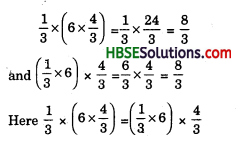We know for any three rational numbers a, b and c, a × (b × c) = (a × b) × c
The multiplication is associative for rational numbers.

Question 8.
Is $$\frac{8}{9}$$ the multiplicative inverse of -1$$\frac{1}{8}$$ ? Why or why not?
Solution:
No, the multiplicative inverse of $$\frac{8}{9}$$ is $$\frac{9}{8}$$ or 1$$\frac{1}{8}$$.
Here, -1$$\frac{1}{8}$$ is negative. Therefore, $$\frac{8}{9}$$ is not multiplicative inverse of -1$$\frac{1}{8}$$.Question 9.
Is 0.3 the multiplicative inverse of 3$$\frac{1}{3}$$ ? Why or why not?
Solution:
0.3 = $$\frac{3}{10}$$
Here, 0.3 × 3$$\frac{1}{3}$$ = $$\frac{3}{10}$$ × $$\frac{10}{3}$$ = 1
We say that a rational number $$\frac{c}{d}$$ is called the reciprocal or multiplicative inverse of another a rational number $$\frac{a}{b}$$ if $$\frac{a}{b}$$ × $$\frac{c}{d}$$ = 1
∴ The result will be yes.

Question 10.
Write :
(i) The rational number that does not have a reciprocal.
Solution:
Zero has no reciprocal.

(ii) The rational numbers that are equal to their reciprocals.
Solution:
1 is equal to its reciprocal, because the reciprical of 1 is 1.
-1 is also equal to its reciprocal because the reciprocal of-1 is -1.
-1 × (-1) = 1

(iii) The Rational number that is equal to its negative.
Solution:
Its value negative.Question 11.
Fill in the blanks :
(i) Zero has ……………. reciprocal.
(ii) The numbers …………….. and ……………. are their own reciprocals.
(iii) The reciprocal of -5 is …………
(iv) Reciprocal of $$\frac{1}{x}$$, where x ≠ 0 is …………
(v) The product of two rational number is always a ……………..
(vi) The reciprocal of a positive rational number is ………………
(iii) $$\frac{1}{-5}$$